# 10-327/Homework Assignment 6

(diff) ← Older revision | Latest revision (diff) | Newer revision → (diff)

### Doing

Solve and submit the following problems.

Problem 1. Problem 1 on page 235 of Munkres' book. (Though following the Errata to Munkres' Book, in part (c) replace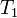$T_1$ by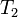$T_2$).

Problem 2. Show that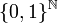$\{0,1\}^{\mathbb N}$ is homeomorphic to the cantor set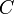$C$.

Problem 3. Show that any function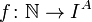$f\colon{\mathbb N}\to I^A$ from the integers into a "cube"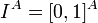$I^A=[0,1]^A$ has a unique continuous extension to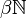$\beta{\mathbb N}$.

Problem 4. Use the fact that there is a countable dense subset within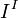$I^I$ to show that the cardinality of$\beta{\mathbb N}$ is greater than or equal to the cardinality of$I^I$.

Problem 5. Show that the cardinality of$\beta{\mathbb N}$ is also less than or equal to the cardinality of$I^I$, and therefore it is equal to the cardinality of$I^I$.

Problem 6. Show that if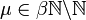$\mu\in\beta{\mathbb N}\backslash{\mathbb N}$ and if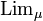$\mbox{Lim}_\mu$ is the corresponding generalized limit, and if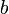$b$ is a bounded sequence and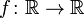$f\colon{\mathbb R}\to{\mathbb R}$ is a continuous function, then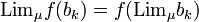$\mbox{Lim}_\mu f(b_k) = f(\mbox{Lim}_\mu b_k)$.

Problem 7. Show that there is no super-limit function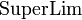$\mbox{SuperLim}$ defined on bounded sequences of reals with values in the reals which has the following 4 properties:

1.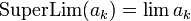$\mbox{SuperLim}(a_k)=\lim a_k$, if the sequence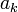$a_k$ is convergent.
2.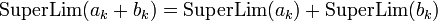$\mbox{SuperLim}(a_k+b_k) = \mbox{SuperLim}(a_k) + \mbox{SuperLim}(b_k)$.
3.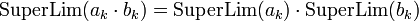$\mbox{SuperLim}(a_k\cdot b_k) = \mbox{SuperLim}(a_k) \cdot \mbox{SuperLim}(b_k)$.
4.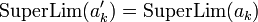$\mbox{SuperLim}(a'_k) = \mbox{SuperLim}(a_k)$, where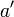$a'$ is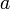$a$ "shifted once":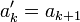$a'_k=a_{k+1}$.

### Due date

This assignment is due at the end of class on Thursday, November 18, 2010.

 Dror's notes above / Student's notes below

Hi Dror, how do we prove some set has smaller cardinality to another set? I mean what do you mean by that? Why in problem 5 it says that the cardinality of a set is less or equal to another set so that the cardinality are the same? Xwbdsb 00:25, 13 November 2010 (EST)

• One way to do this is that if you have a map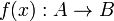$f(x):A \to B$ such that f(x) is 1-1, then$A$ has cardinality less than or equal to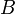$B$. I haven't worked on this question yet, so it may be a red herring, but in general I find the technique useful. John

Also I am wondering what is the super-limit because it wasn't done in class and it is not covered in the book too??Xwbdsb 00:36, 13 November 2010 (EST) I remember that when proving the homeomorphism in question 2 there is another intricate argument for continuity that is not the standard definition in our topology class? Sorry Dror I am sort of lost of what to do for this assignment...Xwbdsb 00:36, 13 November 2010 (EST)

Also what is the generalized limit? I search for this idea in the book but I didn't find anything. I think in the book before we understand Stone-Cech compatification theorem we need to understand what a regular space is and also we need to understand one-point compactification? Isn't Stone-Cech compatification just a special way to compatify the some topological space so that the continuous function with uniquely be extended to the compatification? Xwbdsb 00:58, 13 November 2010 (EST)

• I actually genuinely enjoyed writing this assignment, thinking that I've asked a lovely collection of questions that will challenge you in the positive sense of the word - make you scratch your heads, and struggle a bit, and fight a bit to understand what is going on and eventually get some satisfaction and gain some appreciation of the intricacy and beauty of the subject matter as you understand and solve these problems. So please challenge yourself and think and struggle a bit more. Drorbn 10:32, 13 November 2010 (EST)
• I have a question concerning Problem 7. Perhaps I am misunderstanding it, but if we just define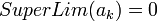$SuperLim(a_k)=0$ for all sequences the 3 properties seem to hold, maybe that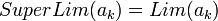$SuperLim(a_k)=Lim(a_k)$ if the sequence does converge should be added? Or maybe just ruling out constant functions is suffient... -John
• Thanks! You are absolutely right. The question has been modified. Drorbn 15:23, 13 November 2010 (EST)

Hi Dror, I understand you want to make it challenging but I think it is also a bit unfair for some students like me. I never learned super-limit function. It is not done in your lecture or discussed in the book. So solving this question totally relies on other background knowledge. I don't have such strong background compared to some other students and I didn't even have a serious lecture on cardinality. I am just hoping since you are asking these kind of questions could you possibly talk about it in class? Trust me Dror it is not that I am not working hard or not trying to struggle but my background is not even enough for me to understand the question. For example I don't know what super-limit function is. I couldn't find it in Munkres book I couldn't find it by googling? Are you talking about limsup?

And what is the generalized definition of limit? I cannot find the definition anywhere.Xwbdsb 10:01, 14 November 2010 (EST)

• In the question about super-limits you are asked to prove that "super-limits", objects with the given properties, do not exist. It is not surprising they are not in the literature. Cardinalities are covered in MAT246, which is a pre-requisite to this class, I believe. I'll say more about generalized limits in the coming class tomorrow. Drorbn 10:37, 14 November 2010 (EST)
• Munkres Chapter 1 is all about set theory. In particular sections 6,7 and 9 have discussions about cardinality, this may be a good resource if you are having difficulties with the ideas. - John

• A quick question (and I hope it isn't stupid...), but I'm not sure what "A" is when you talk about the cube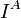$I^A$. What product of the unit interval are you talking about? --Wesley 12:15, 14 November 2010 (EST)
•$A$ is any set whatsoever. So the "cube"$I^A$ is finite dimensional if$A$ is finite, but it is infinite dimensional if$A$ is infinite. Drorbn 13:28, 14 November 2010 (EST)
• Is it true that for question 6 the function f has to be bounded? Since even though b is a bounded sequence but f(b_k) might not be bounded so that the generalized limit is not defined. given that f is continuous. suppose f=1/x and b_k = 1/k. Xwbdsb 22:12, 15 November 2010 (EST)
• The function in the example you gave is not continuous; it not even defined at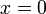$x=0$. Drorbn 07:10, 16 November 2010 (EST)
• In problem 1 part c, the errata to Munkres says that should be Hausdorff.
• Thanks! Drorbn 07:10, 16 November 2010 (EST)
• Does the set of all bounded sequences has the same cardinality as the reals?Xwbdsb 15:00, 16 November 2010 (EST)

Should we assume that {0,1} has the discrete topology and that {0,1}^N has the corresponding product topology and should we also assume that C has the subspace topology that it inherits from R? Thank you.

• Yes and yes. Drorbn 06:59, 17 November 2010 (EST)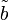$\tilde b$ has to be bounded because$\beta{\mathbb N}$ is compact. Ian 18:54, 17 November 2010 (EST)